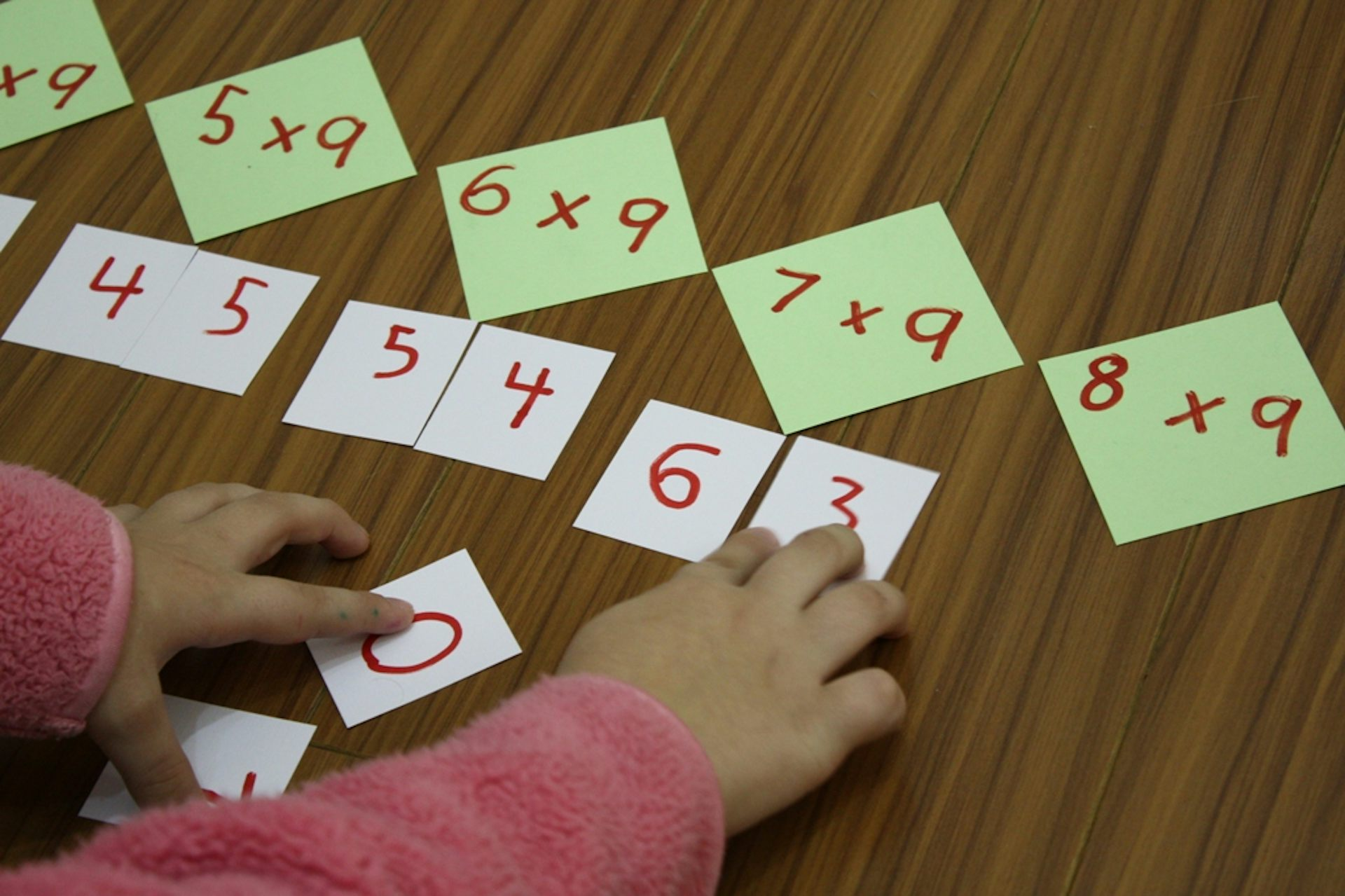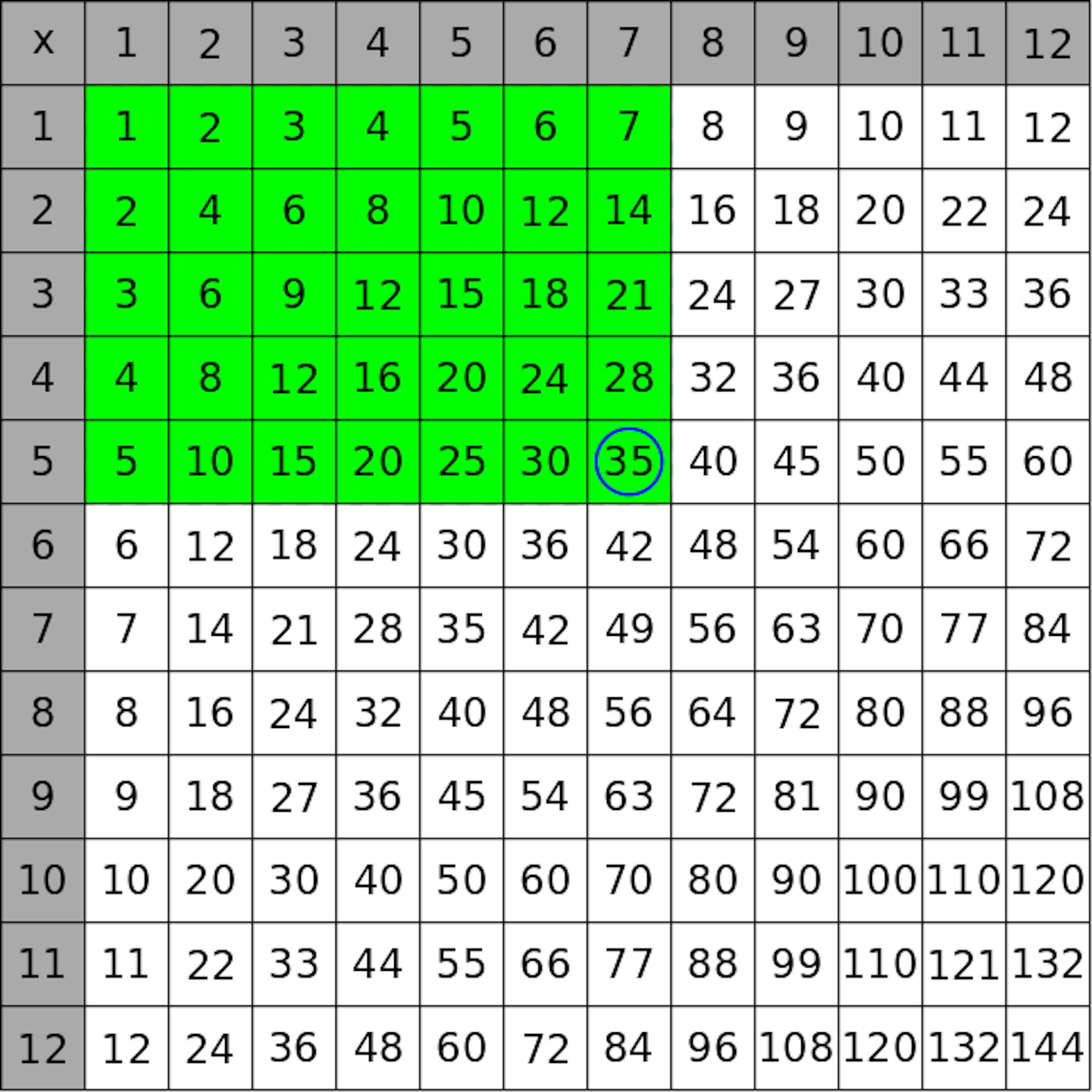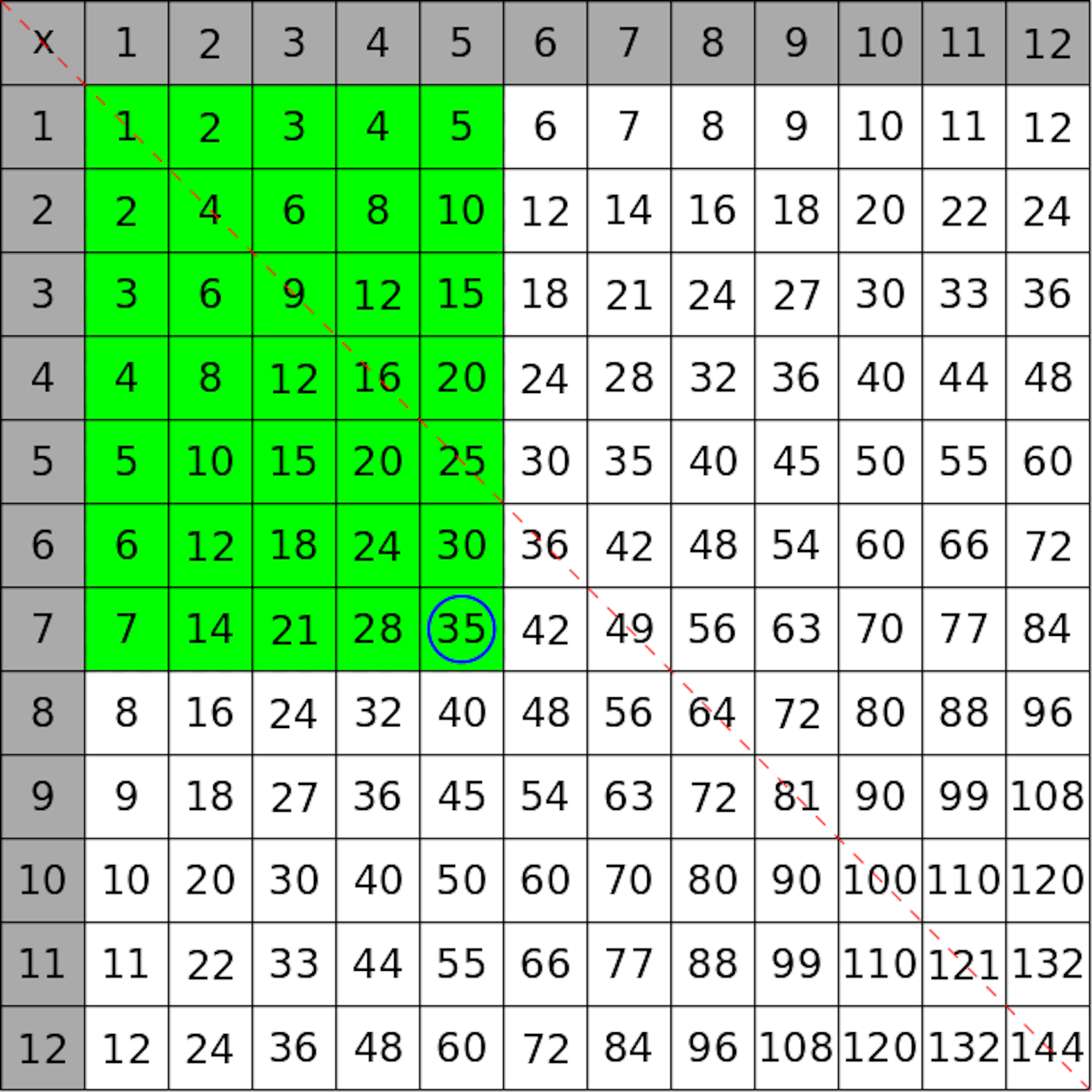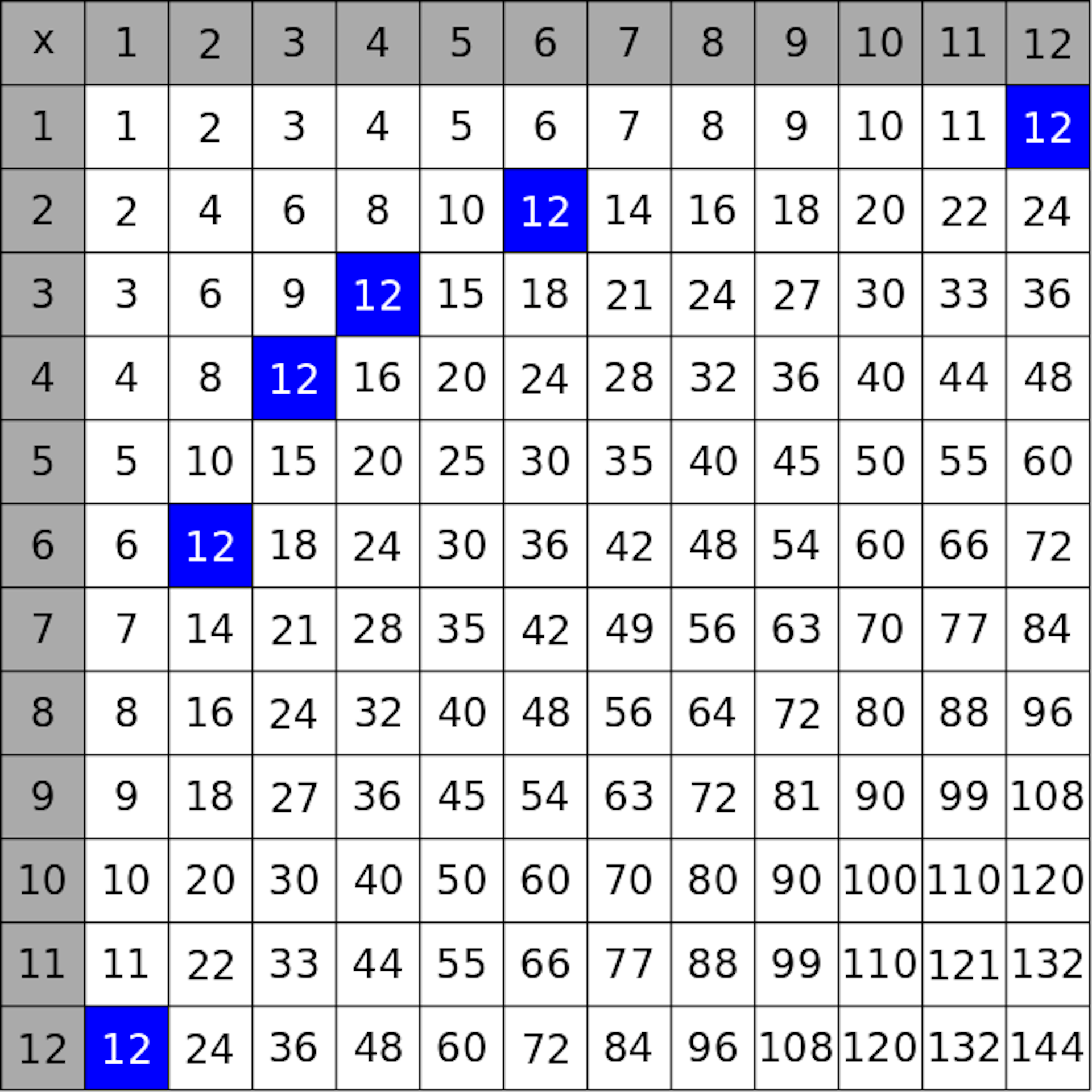# A little number theory makes the times table a thing of beauty

Sunday, Jun 7, 2015, 11:16 PM | Source: The Conversation

By Anita Ponsaing

# A little number theory makes the times table a thing of beautyDoing the 9 times table. Flickr/Jimmie, CC BY-NC-SA

Most people will probably remember the times tables from primary school quizzes. There might be patterns in some of them (the simple doubling of the 2 times table) but others you just learnt by rote. And it was never quite clear just why it was necessary to know what 7 x 9 is off the top of your head.

Well, have no fear, there will be no number quizzes here.

Instead, I want to show you a way to build numbers that gives them some structure, and how multiplication uses that structure.

## Understanding multiplication

Multiplication simply gives you the area of a rectangle, if you know the lengths of the sides. Pick any square in the grid, (for example, let's pick the 7th entry in the 5th row) and colour a rectangle from that square to the top left corner.A rectangle of size 5 × 7 in the multiplication table.

This rectangle has length 7 and height 5, and the area (the number of green squares) is found in the blue circle in the bottom right corner! This is true no matter which pair of numbers in the grid you pick.

Now let's take this rectangle and flip it around the main diagonal (the red dotted line).The same rectangle, flipped.

The length and height of the rectangle have swapped, but the area hasn't changed. So from this we can see that 5 × 7 is the same as 7 × 5. This holds true for any pair of numbers — in mathematics we say that multiplication is commutative.

But this fact means that there is a symmetry in the multiplication table. The numbers above the diagonal line are like a mirror image of the numbers below the line.

So if your aim is to memorise the table, you really only need to memorise about half of it.

## The building blocks of numbers

To go further with multiplication we first need to do some dividing. Remember that dividing a number just means breaking it into pieces of equal size.

12 ÷ 3 = 4

This means 12 can be broken into 3 pieces, each of size 4.

Since 3 and 4 are both whole numbers, they are called factors of 12, and 12 is said to be divisible by 3 and by 4. If a number is only divisible by itself and 1, it is called a prime number.

But there's more than one way to write 12 as a product of two numbers:

12 × 1

6 × 2

4 × 3

3 × 4

2 × 6

1 × 12

In fact, we can see this if we look at the multiplication table.The occurrences of 12 in the multiplication table.

The number of coloured squares in this picture tells you there are six ways you can make a rectangle of area 12 with whole number side lengths. So it's also the number of ways you can write 12 as a product of two numbers.

Incidentally, you might have noticed that the coloured squares seem to form a smooth curve — they do! The curve joining the squares is known as a hyperbola, given by the equation a × b = 12, where 'a' and 'b' are not necessarily whole numbers.

Let's look again at the list of products above that are equal to 12. Every number listed there is a factor of 12. What if we look at factors of factors? Any factor that is not prime (except for 1) can be split into further factors, for example

12 = 6 × 2 = (2 × 3) × 2

12 = 4 × 3 = (2 × 2) × 3

No matter how we do it, when we split the factors until we're left only with primes, we always end up with two 2's and one 3.

This product

2 × 2 × 3

is called the prime decomposition of 12 and is unique to that number. There is only one way to write a number as a product of primes, and each product of primes gives a different number. In mathematics this is known as the Fundamental Theorem of Arithmetic.

The prime decomposition tells us important things about a number, in a very condensed way.

For example, from the prime decomposition 12 = 2 × 2 × 3, we can see immediately that 12 is divisible by 2 and 3, and not by any other prime (such as 5 or 7). We can also see that it's divisible by the product of any choice of two 2's and one 3 that you want to pick.

Furthermore, any multiple of 12 will also be divisible by the same numbers. Consider 11 x 12 = 132. This result is also divisible by 1, 2, 3, 4, 6 and 12, just like 12. Multiplying each of these with the factor of 11, we find that 132 is also divisible by 11, 22, 33, 44, 66 and 132.

It's also easy to see if a number is the square of another number: In that case there must be an even number of each prime factor. For example, 36 = 2 × 2 × 3 × 3, so it's the square of 2 × 3 = 6.

The prime decomposition can also make multiplication easier. If you don't know the answer to 11 × 12, then knowing the prime decomposition of 12 means you can work through the multiplication step by step.

11 x 12

= 11 x 2 × 2 × 3

= ((11 x 2) × 2) × 3

= (22 × 2) × 3

= 44 × 3

= 132

If the primes of the decomposition are small enough (say 2, 3 or 5), multiplication is nice and easy, if a bit paper-consuming. Thus multiplying by 4 (= 2 x 2), 6 (= 2 x 3), 8 (= 2 x 2 x 2), or 9 (= 3 x 3) doesn't need to be a daunting task!

For example, if you can't remember the 9 times table, it doesn't matter as long as you can multiply by 3 twice. (However this method doesn't help with multiplying by larger primes, here new methods are required – if you haven't seen the trick for the 11 times tables watch this video).

So the ability to break numbers into their prime factors can make complicated multiplications much simpler, and it's even more useful for bigger numbers.

For example, the prime decomposition of 756 is 2 x 2 x 3 x 3 x 3 x 7, so multiplying by 756 simply means multiplying by each of these relatively small primes. (Of course, finding the prime decomposition of a large number is usually very difficult, so it's only useful if you already know what the decomposition is.)

But more than this, prime decompositions give fundamental information about numbers. This information is widely useful in mathematics and other fields such as cryptography and internet security. It also leads to some surprising patterns – to see this, try colouring all multiples of 12 in the times table and see what happens. I'll leave that for homework.Anita Ponsaing, Research Associate in Mathematics, University of Melbourne

This article is republished from The Conversation under a Creative Commons license. Read the original article.